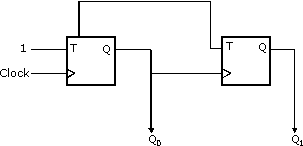# GATE | GATE CS 2010 | Question 65

In the sequential circuit shown below,if the initial value of the output Q1Q0 is 00,what are the next four values of Q1Q0?(A) 11, 10, 01, 00
(B) 10, 11, 01, 00
(C) 10, 00, 01, 11
(D) 11, 10, 00, 01

Explanation: We have t flip flop

Truth table of t flip flop

 t q 0 q 1 q’

So q0 always inverted as t=1 always

So 1)q10=1

2)q0 =0

3)q0 =1

4)q0 =1

For q1 also t=1 always but clock is so we have to observe positive edge of clock I.e. when is q0 going from 0 -> 1

1)q1 =1

2)q1 =1

3)q1 =0

4)q1 =0

So final combination q0q1->(11,10,01,00) Ans (A)

Quiz of this Question

My Personal Notes arrow_drop_up
Article Tags :

Be the First to upvote.

Please write to us at contribute@geeksforgeeks.org to report any issue with the above content.